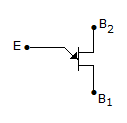# Electronics and Communication Engineering - Power Electronics

### Exercise :: Power Electronics - Section 7

21.

In a certain inverter circuit each thyristor carries 26.67 A for 60°, 13.33 A for 120° and zero A for 180° of the cycle. The rms current of thyristor is

 A. 20 A B. 13.33 A C. 6.67 A D. 3.33 A

Explanation:

No answer description available for this question. Let us discuss.

22.

The factor η for a UJT is about

 A. 0.1 B. 0.2 C. 0.6 D. 0.9

Explanation:

No answer description available for this question. Let us discuss.

23.

The symbol in figure is forA. UJT B. PUT C. Shockley diode D. TRIAC

Explanation:

No answer description available for this question. Let us discuss.

24.

In a single phase full converter fed by a source having inductance, the number of thyristors conducting during overlap is

 A. 1 B. 2 C. 3 D. 4

Explanation:

No answer description available for this question. Let us discuss.

25.

The cut in voltages for germanium and silicon diodes respectively as

 A. 0.3 V and 0.7 V B. 0.7 V and 0.3 V C. 0.6 V and 0.9 V D. 0.5 V and 0.7 V